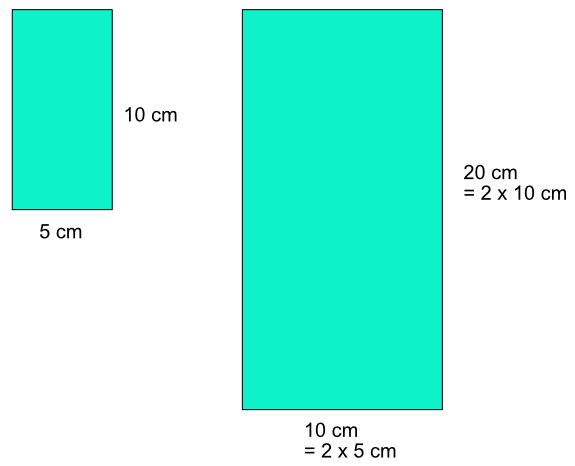Relationship of Length, Area and Volume

# Relationship of Length, Area and Volume

GCSE(H),

A line is drawn that is 5cm long. Applying a scale factor of 2, the line becomes 2 x 5cm = 10cm long.

If a rectangle is 10 cm by 5cm, the are oaf the rectangle is 5 x 10 = 50 cm2.

If the linear lengths of the rectangle are doubled, then the area is calculated as
20 x 10
= 2 x 10 x 2 x 5
= 4 x 50
which indicates that the area of the rectangle is 22 times larger.If the linear lengths of the rectangle are increased by a scale factor of 3, then the area increases by a scale factor of 32. For similar figures, the area of a shape increases by the square of the scale factor.

Volumes increase in a similar way, but as a cube of the scale factor. If a cuboid measuring 2, 3 and 4cm has its linear measurement increased by a scale factor of 3.

The original volume is 2 x 3 x 4 = 24 cm2.

The enlarged volume is 6 x 9 x 12 cm3
= (3 x 2) x (3 x 3) x (3 x 4) cm3
= 33 x 2 x 3 x 4 cm3
= 33 x 24 cm3

## Examples

1. A cuboid has a volume of 22 cm3. A similar cuboid has a length that is 1.5 times the size of the original. What is the volume of the similar cuboid? Give the answer to 1 decimal place.

The volume of a shape increases by a cube of the scale factor. The volume of the enlarged cuboid is 22 x 1.52 = 74.25

To 1 dp this is 74.3cm3

2. A rectangle, A, has an area of 100cm2. A similar shape, B, has an area of 200cm2. What is the scale factor to map A onto B? Give the answer correct to 2 decimal places.